## 确定执行的优先级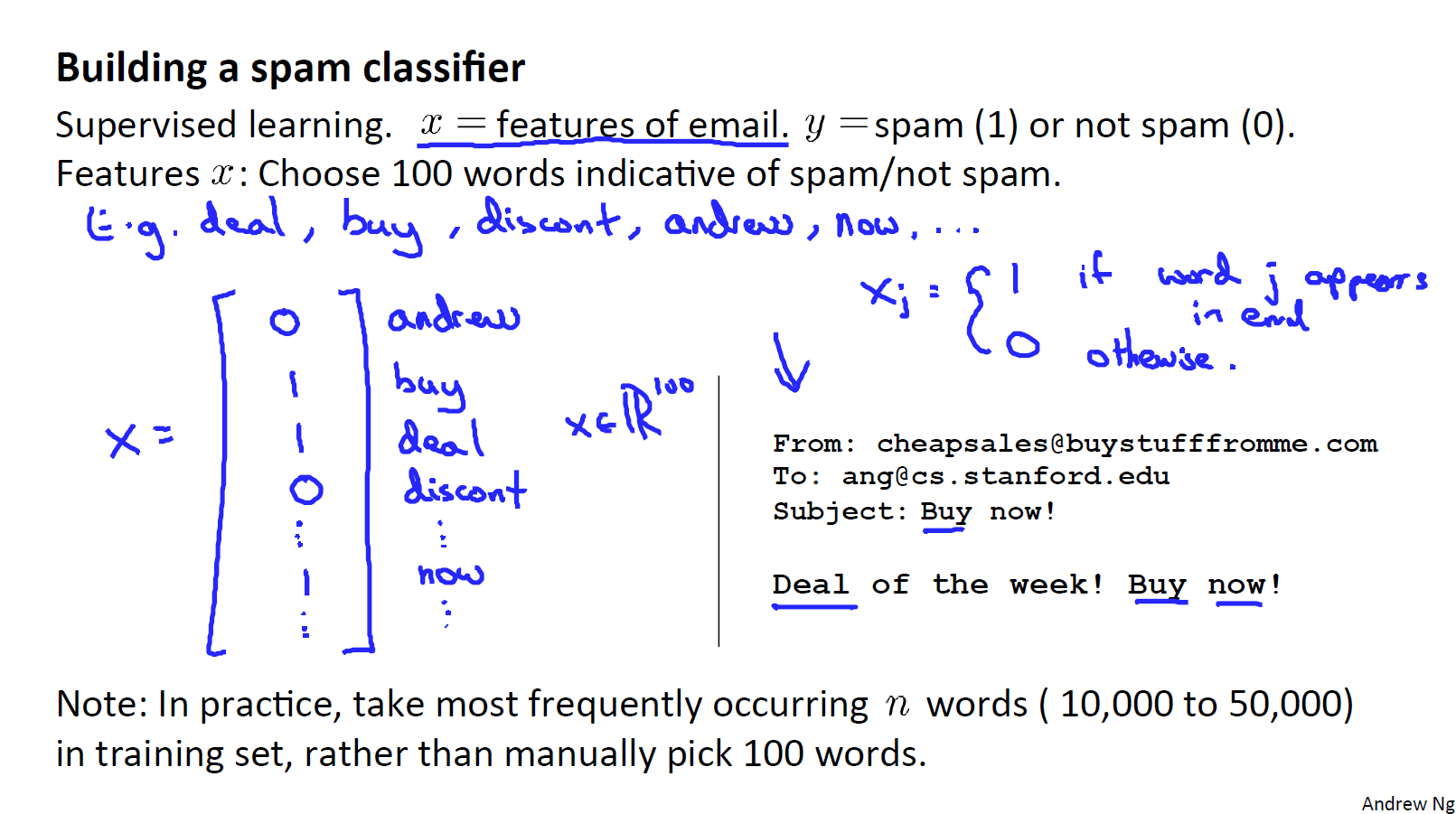• 收集更多的数据
• 增加更复杂的特征（比如邮件头）
• 开发更复杂的算法来鉴定错误拼写

## 误差分析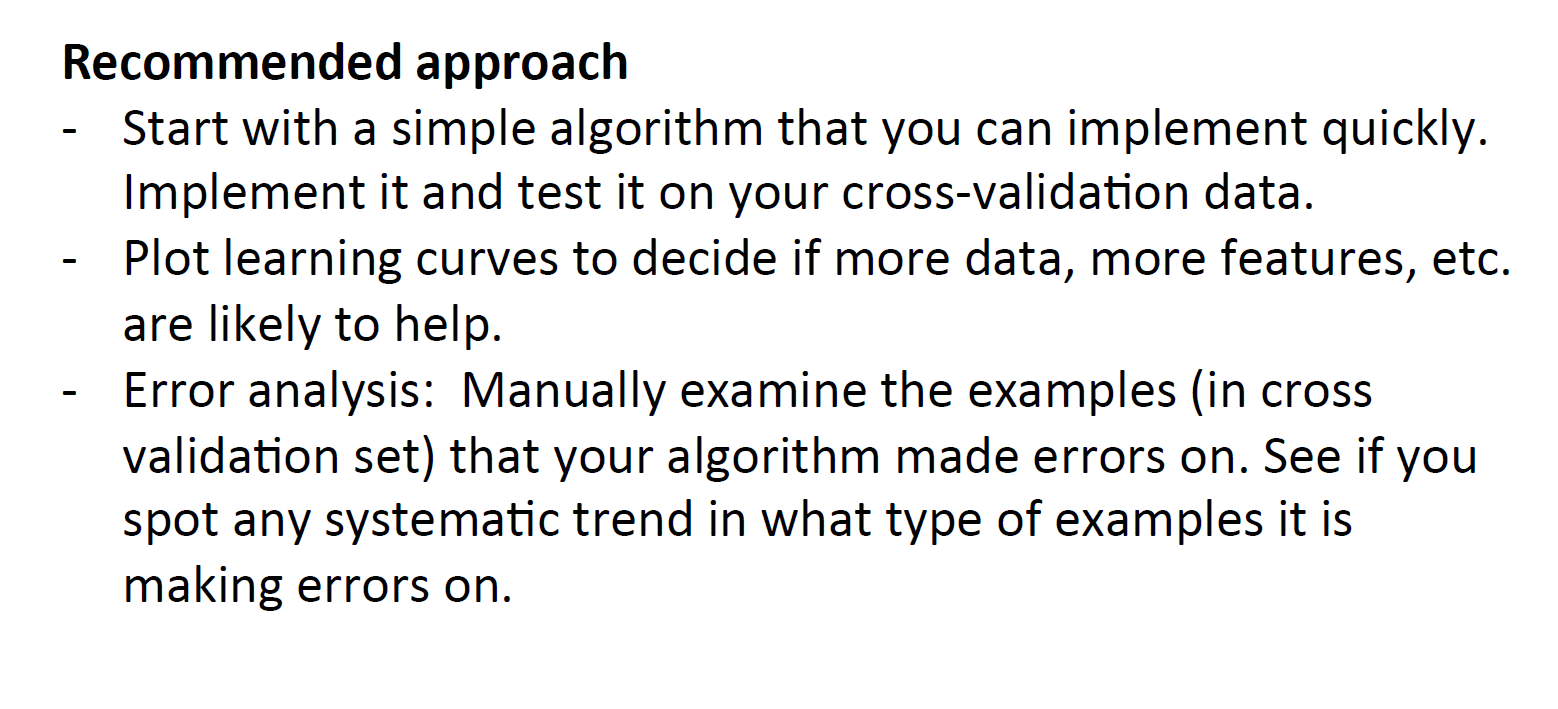## 偏斜类问题的误差评估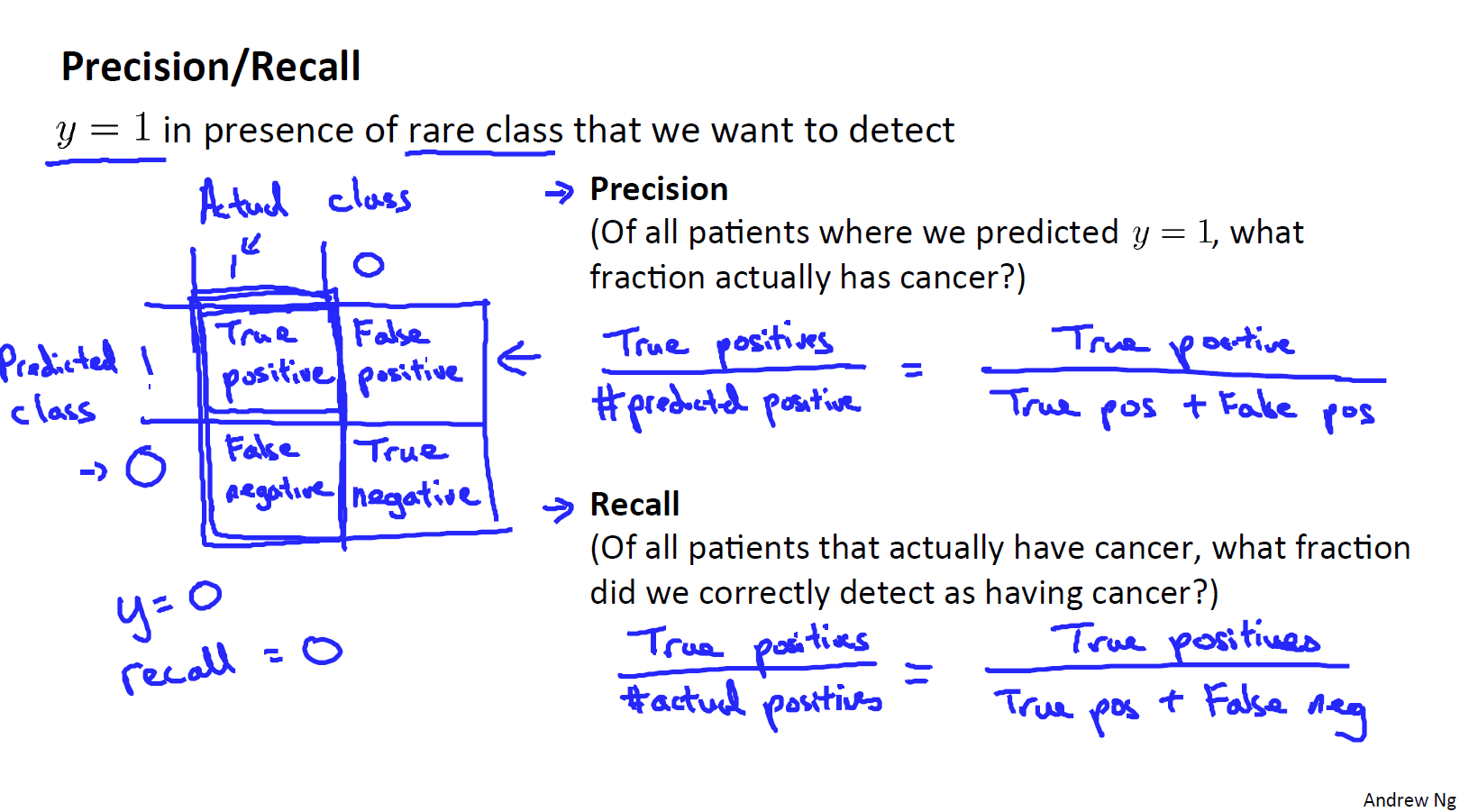\frac{True Positives}{Predicted Positive} = \frac{True Positive}{True Positive+False Positive}

\frac{True Positives}{Actual Positive} = \frac{True Positive}{True Positive+False Negative}

## 查准率和召回率的权衡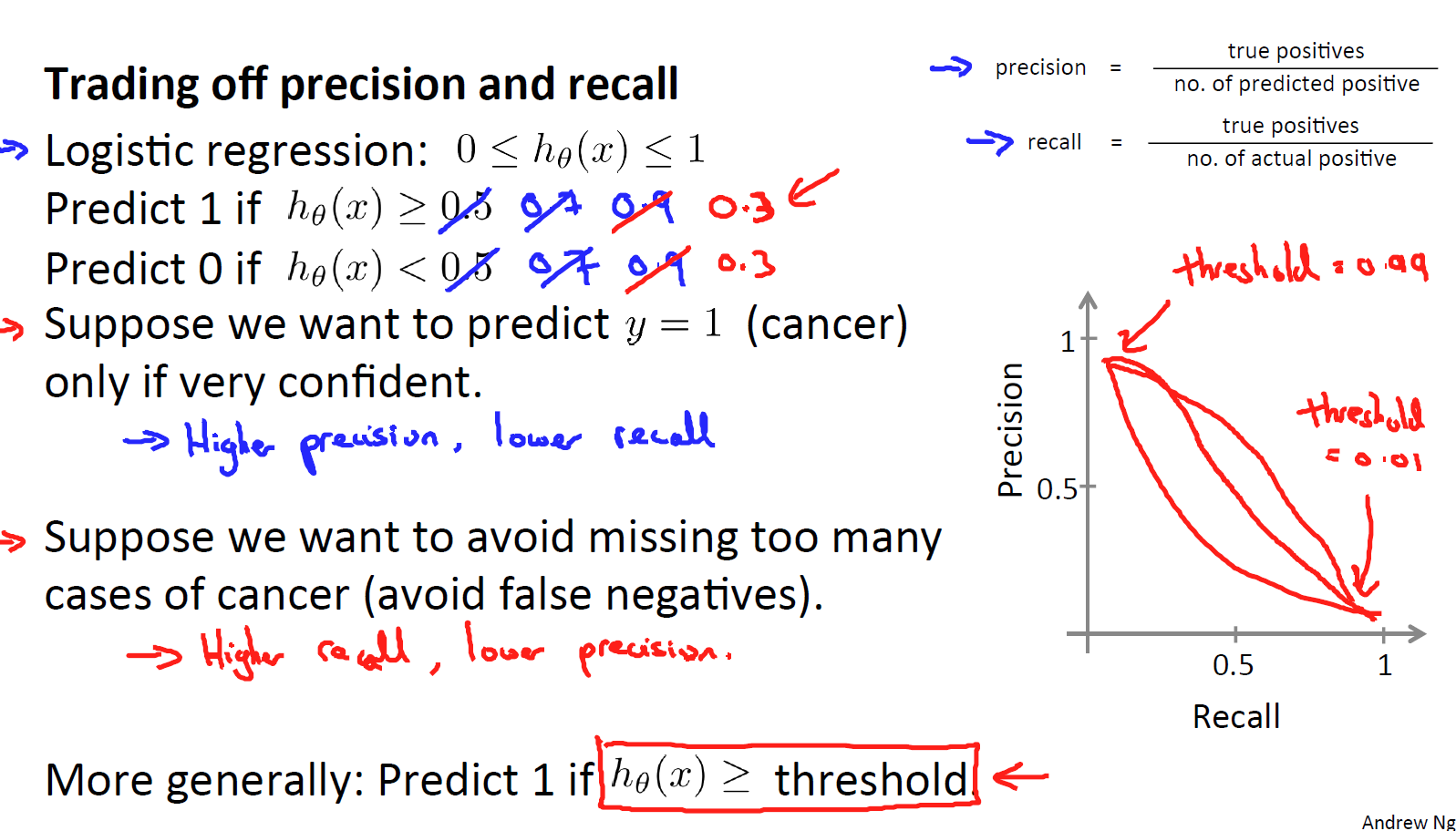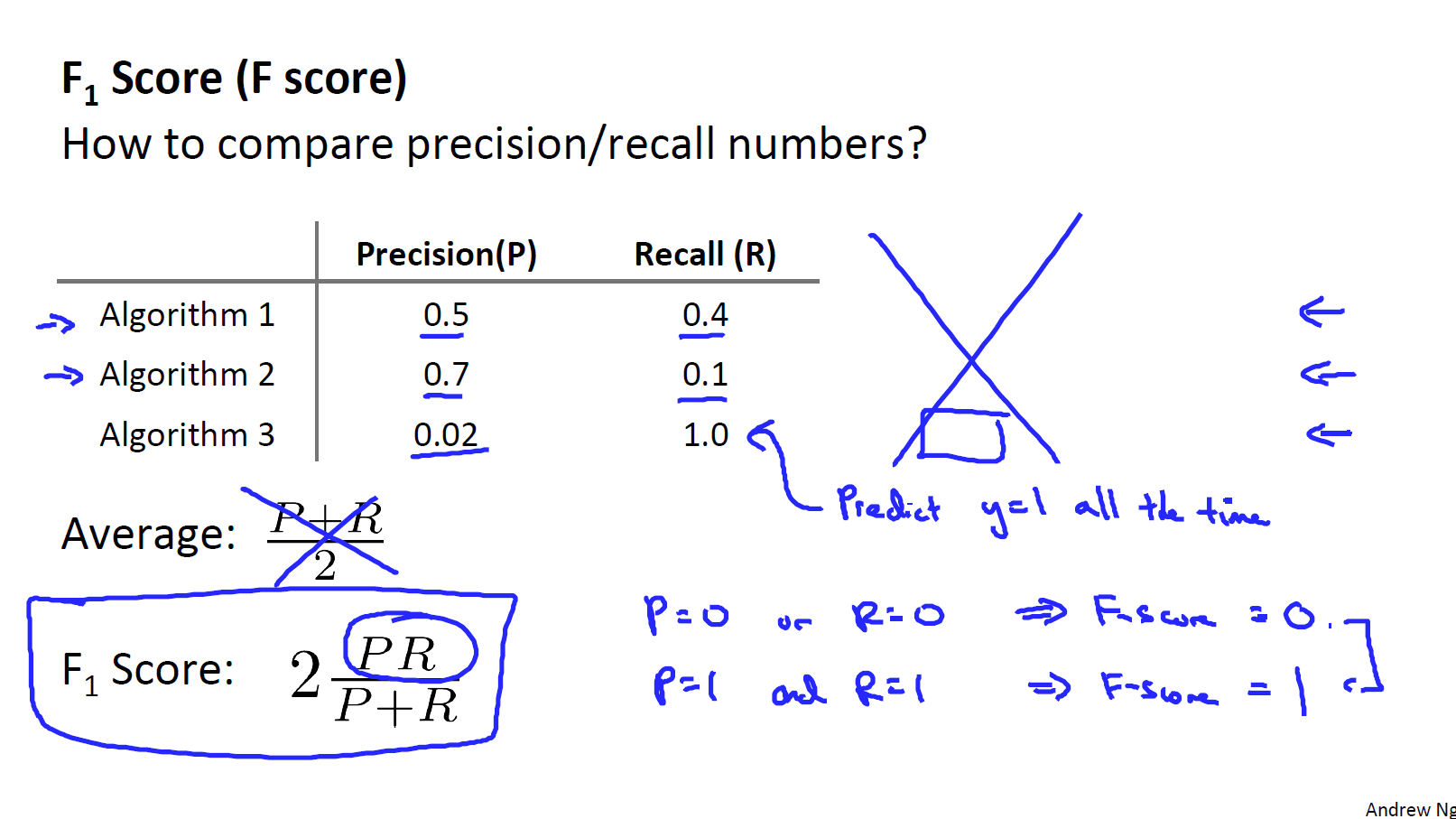F Score = 2\frac{PR}{P+R}